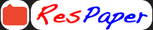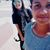Trending ▼   ResFinder# Class 12 ISC Prelims 2018 : Mathematics (Christ Vidyanikethan ICSE, Irinjalakuda, Thrissur)

4 pages, 51 questions, 39 questions with responses, 68 total responses,10Aleena Mavely +Fave Message ProfileTimelineUploads
 Home > aleena000 >   F Also featured on: School Page iscFormatting page ...

C H R I S T V D Y A N I K E T H A N F IR S T M O D E L E X A M IN A 1 O N 2 0 1 7 M A B E M I b rs Jm e : j M C d id a t e s an C a n a re a A n d a T hey m e q u I n te r n a e s t io n s S e c tio n m in u te s fo r o n y d tw s been a c h o ic e I n te r n a c h o ic e h a A w o r k in T h e in te n d e d m a M a B a n d C q u e s tio n s fr o m S e c tio n A pta S e c tio n B O R S e c tio n C p r o v id e d in t h r e e q u e s tio n s o f 4 m q u e s tio n s o f 6 m a r k s h a s b e e n p r o v id e d in 2 I n te r n a n d ea c a r ks u d in g r o u g in c a s a n d a d ja c e n t to t h e r e s t o f th e a n s w e r r k s fo r q u e s tio n s o r p a r ts o f q u e s tio n s a r e g iv t h e m a t ic a l ta b es a n d g ra p h p a p e r a re h ea c h q u e s tio n s o f 4 m a r k s b e e n p r o v id e d in 2 u e s t io n s o f 4 m a r k q s b e d o n e o n t h e s a m e s h e e t gh w o rk sha s p ro v en ea c h ea c h in b r a c k e t s [] id e d 11 0 2 _ su c der h th a t A 5 m l o n g i s i n g a g a in s t a r a te o f 2 c m /s H c o n ta m s ow 10 b a lls , 2 _ kA w a ll th e b a g w h m a rke d w . (W h e r e T h e b o tto fa s t is its h e ig h t o eac 21 n m o ' 's th e id e n tity 2 m a tf ix o f th e la d d e r is p u lle d a lo n th e r o u d n aw g g g w h e n th e fo o t o f th e la d d e r is 4 th e w a ll d e c r e a s in X < o x 0 = , o n e o 4 a m y o m th e aw a tin u o u s at x = O , d e te rm in e th e 20 1 s u c c e s s iv e v a lu e o w a ll y fr o m th e ly w ith . co n - f o rd e r 2 ) f th e d ig its 0 to 9 If fo w b a lls a r e u a w n h a t is tb e p r o b a b ility th a t n o n e is m a r k e d w i e d ig it 0 ' ? ith 10 0 m o C , a rks d in g th e p a p e r rea t s ta t d u r in g th is tim e s is ts o f t h r e e s e c tio n s A E ith e r fr o B n 1 5 n a u st n o l c h o ic e h a a n S e c tio d d it io T h e q u e s t io n p a p e r c o n d id a t e s a r e 11e q u ir e d to a e r n a S e c t io l o w ] fa Scanned by CamScannerFormatting page ...

Top Contributors
to this ResPaperVishnu Shankar(9)Anup Kumar(8)Jasmeet Bindra(8)Vaibhav Lakhani(6)Formatting page ...Formatting page ...